# How to multiply long numbers

Assuming we have a multiplication of n-bit-numbers already available.
Other than typical compiled languages, which assume that the multiplication result fits into the same size as the two factors, we should take a closer look.
Unsigned n-bit-numbers as factors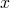and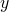fullfill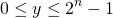So we have for the product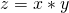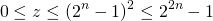So we should be prepared for a 2n-bit long result. Assembly language provides for this. In Java we are kind of running out of luck, because we have the 64-bit-long as the largest possible value, so we cannot make use of the 64-bit capabilities of our CPU by getting a 128-bit-result. Even worse we do not have unsigned primitive types. For addition and subtraction it is possible to cheat by assuming that the longs are unsigned, because the addition for signed and unsigned numbers is almost the same, but for multiplication this is slightly more complex.

In C we do have better chances, but we need to go compiler and OS-specific to some extent. gcc on 64-bit platforms has a type __uint128_t and we can hope that something like this
 __uint128_t mul(unsigned long long x, unsigned long long y) { __uint128_t xx = (__uint128_t) x; __uint128_t yy = (__uint128_t) y; __uint128_t z = xx*yy; return z; } 
is optimized by the compiler to one assembly language instruction that multiplies two unsigned 64-bit-integers into one unsigned 128-bit integer.

Multiplication of longer numbers is achieved by just assuming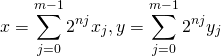with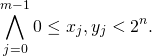Now the product iswhere each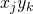summand needs to be split into a lower and an upper part such that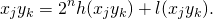All these half products need to be added with propagating the carry bits to the next higher level.
This can be sorted out and done with a lot of additions with carry-bit, finally giving the desired result.

For reasonably short numbers this is a good approach, if it is done well, but it can be replaced by better algorithms for larger numbers.

The most obvious next step is to move to the Karatsuba algorithm.
Assuming we have two factors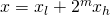andthe naïve approach of having to calculate the four products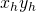,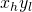,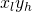,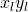can be replaced by calculation of only three products,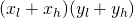,, because what is actually needed is the sum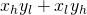which can be obtained by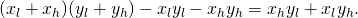That can be iterated by subdividing the numbers subsequently into halves until a size is reached that can be handled well enough by the naïve approach.
An issue that needs to be observed is that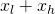and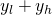need one bit more than their summands.

For even larger numbers the so called Toom-Cook algorithm, an extension of the idea of the Karatsuba-algorithm for more than two summands, can be useful and for numbers larger than that the Schönhage-Strassen multiplication proves to be faster. It is implemented in some libraries for long integer arithmetic, like GMP. Since around 2007 this can be improved by using the Fürer algorithm which is assymptotically faster, but I do not know of any useful implementations and for practical purposes the improvement does not seem to be very relevant.